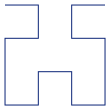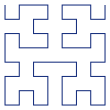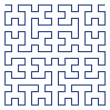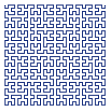# Visualising IP Geolocation

2009-09-05

I'm spending a fair bit of my time working on a project that uses an IP geolocation database to map internet addresses to countries as part of a security survey. There are a number of these location databases available, but comparing their quality and coverage is not trivial, so selecting one to use is hard. I recently decided to spend a few hours looking at the problem, and got hopelessly side-tracked into visualising the databases using the Hilbert curve. The result is the Hilbert Explorer, a mapping of the geographical location of IP addresses onto the Hilbert Curve. You should have a play with it before reading the rest of this post.

## The Hilbert Curve - a (very) brief introduction

The Hilbert Curve is a space-filling curve that is usually produced iteratively, with the N-th step in the iteration referred to as the "order N" curve. Here are orders 1 to 5:N=1N=2N=3N=4N=5

To translate from one order to the next, we simply replace U-shapes like the the N=1 diagram with Y-shapes like the N=2 diagram. So, in the N=1 diagram there is a single U to be replaced, in the N=2 diagram there are 4 U-shapes (two at the top, oriented left and right, and two at the bottom oriented down). Each subsequent order has 4 times the number of U shapes the previous one had, so for N=3 we have 16 replacements to do, and so on and so forth.

Mathematicians are interested in the behaviour of the limit curve as N approaches infinity - luckily the properties of the curve that are interesting to computer scientists manifest well short of that. For the purposes of this post, we can view the order-N curve simply as a way to lay out a sequence of 2**2N items on a plane, with the rather interesting property that items that are near each other in the sequence are also near each other on the plane:

The recursive construction above is a nice way to explain the curve, but doesn't lead to an efficient way to actually draw it. For this I turned to Henry S. Warren's wonderful Hacker's Delight, one of those books that I return to again and again. If you don't already own a copy, just buy it - you won't be disappointed. All the images in this post and in the Explorer were drawn with PyCairo using the algorithm for calculating co-ordinates from the distance along the curve given in section 14.4 of this book.